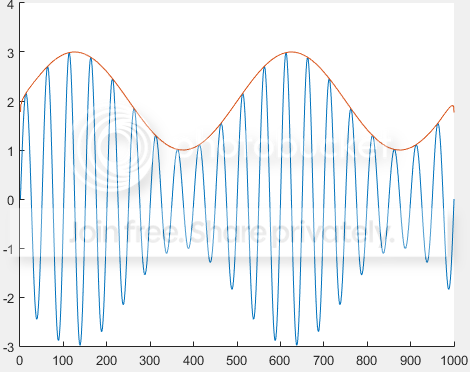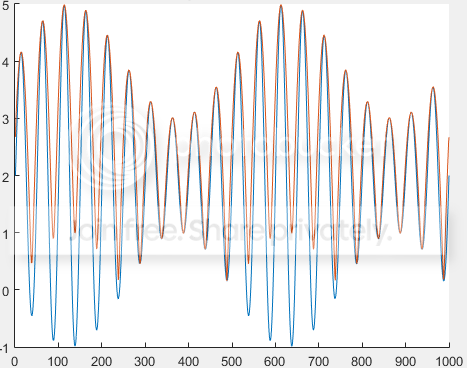# Envelope detector for non-zero mean signal (Matlab)

I used hilbert transform to get an envelope signal.

envelope = abs(hilbert(inputSignal))


This works for zero-mean signal:But, for nonzero-mean case, the output is not what expected.If I do not want to remove the mean level of signal,
How can I extract the same envelope as in the first case?

You can calculate the mean of your nonzero signal, then substract it to the non-zero mean signal and calculate the envelope as you did before.

That will give you the envelope of the signal for the zero mean signal, but if you want to recover the envelope for the non-zero mean signal, then you just have to add to this envelope the initially calculated mean.

• I do not want to do anything with the mean level because it may be non constant over time. I think it has to be the direct way to go, but I still cannot figure it out. – pakornosky Aug 24 '16 at 13:37
• I have read that there is a function called envelope in matlab, may be useful for you de.mathworks.com/help/signal/ref/envelope.html – Josu Etxezarreta Martinez Aug 24 '16 at 13:45

A hilbert filter is, at the end of the day, a complex bandpass that filters out the negative-frequency half of your double-sided spectrum.

A "mean" is the zero-frequency component of the signal. Whether that should, in theory, be erased by a Hilbert filter, halved or left alone might be up for discussion. In practice, it's usually filtered away (which is a mathematical necessity considering you can't have infinitely sharp filters with finite lengths).

In other words, your Hilbert is doing what it should. If you really need your DC component, as Josu recommended, estimate that and add it back up (you can at least not make a phase error, here).

I'm not even sure I'd use a hilbert filter for envelope detection in your case, to be honest. It's incomplete, to say the very least – the envelope of a signal is $\sqrt{x^2 + \bar x^2}$, $\bar x$ being the hilbert transform of the signal $x$, and you're only calculating $|\bar x|$. Notice that $\bar x$ is complex and hence $\bar x^2$ might be negative!

• In Matlab, hilbert(x) actually returns x+j*y, where y is the Hilbert transform of x. – MBaz Aug 24 '16 at 16:17
• aaaah, @MBaz, thanks. yeah, then, that is a envelope detector :) – Marcus Müller Aug 24 '16 at 17:05
• I now get that I cannot avoid the step of remove/envelope-detection/compensate the mean of signal if I use Hilbert transform. Could you suggest another way instead of Hilbert? The more direct method to achieve this job and has nothing to deal with mean component. – pakornosky Aug 24 '16 at 19:57